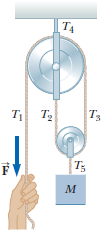Problem: An object of mass M is held in place by an applied force F and a pulley system as shown below. The pulleys are massless and frictionless.(a) Draw diagrams showing the forces on each pulley.(b) Find the tension in each section of rope, T1, T2, T3, T4, and T5(c) Find the magnitude of F.

90% (97 ratings)
Problem Details

An object of mass M is held in place by an applied force F and a pulley system as shown below. The pulleys are massless and frictionless.
(a) Draw diagrams showing the forces on each pulley.
(b) Find the tension in each section of rope, T1, T2, T3, T4, and T5
(c) Find the magnitude of F.